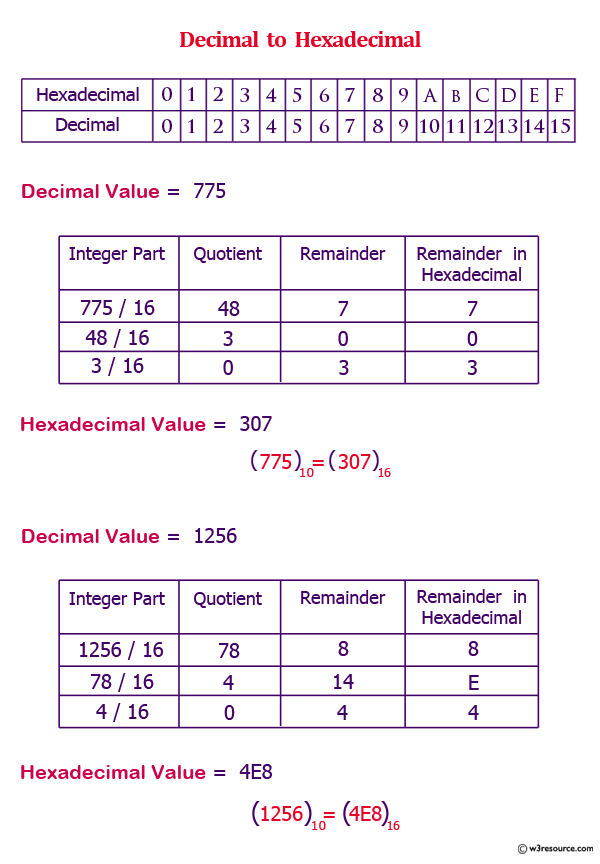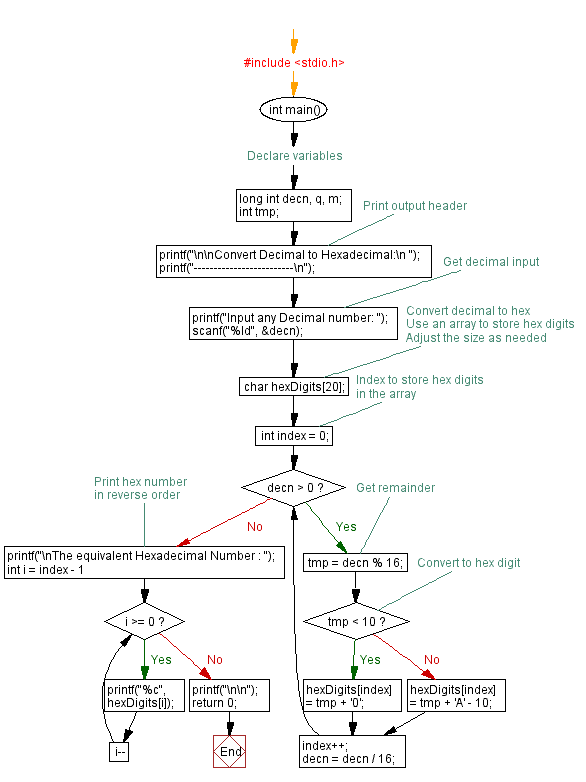﻿ C Program: Convert a decimal number to hexadecimal - w3resource# C Exercises: Convert a decimal number to hexadecimal

## C For Loop: Exercise-55 with Solution

Write a program in C to convert a decimal number to hexadecimal.

Pictorial Presentation:Sample Solution:

C Code:

``````#include <stdio.h>

void main()
{
long int decn,rmd,q,dn=0,m,l;
int i=1,j,tmp;
char s;

printf("-------------------------\n");

printf("Input  any Decimal number: ");
scanf("%ld",&decn);
q = decn;
for(l=q;l>0;l=l/16)
{
tmp = l % 16;
if( tmp < 10)
tmp =tmp + 48; else
tmp = tmp + 55;
dn=dn*100+tmp;
}
printf("\nThe equivalent Hexadecimal Number : ");
for(m=dn;m>0;m=m/100)
{
s=m % 100;
printf("%c",s);
}
printf("\n\n");
}
```
```

Sample Output:

```Convert Decimal to Hexadecimal:
-------------------------
Input  any Decimal number: 79

The equivalent Hexadecimal Number : 4F
```

Flowchart:C Programming Code Editor:

Improve this sample solution and post your code through Disqus.

What is the difficulty level of this exercise?

Test your Programming skills with w3resource's quiz.

﻿